# ML Aggarwal Class 10 Solutions for ICSE Maths Chapter 17 Mensuration Ex 17.1

## ML Aggarwal Class 10 Solutions for ICSE Maths Chapter 17 Mensuration Ex 17.1

ML Aggarwal Class 10 Solutions for ICSE Maths Chapter 17 Mensuration Ex 17.1

Take π = $$\\ \frac { 22 }{ 7 }$$ unless stated otherwise.

Question 1.
Find the total surface area of a solid cylinder of radius 5 cm and height 10 cm. Leave your answer in terms of π.
Solution:Question 2.
An electric geyser is cylindrical in shape, having a diameter of 35 cm and height 1.2 m. Neglecting the thickness of its walls, calculate
(i) its outer lateral surface area,
(ii) it’s capacity in litres.
Solution: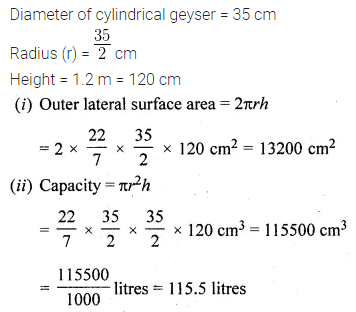Question 3.
A school provides milk to the students daily in cylindrical glasses of diameter 7 cm. If the glass is filled with milk up to a height of 12 cm, find how many litres of milk is needed to serve 1600 students.
Solution:Question 4.
In the given figure, a rectangular tin foil of size 22 cm by 16 cm is wrapped around to form a cylinder of height 16 cm. Find the volume of the cylinder.Solution:Question 5.
(i) How many cubic metres of the soil must be dug out to make a well 20 metres deep and 2 metres in diameter?
(ii) If the inner curved surface of the well in part (i) above is to be plastered at the rate of Rs 50 per m2, find the cost of plastering.
Solution: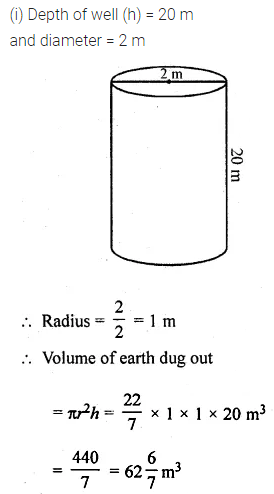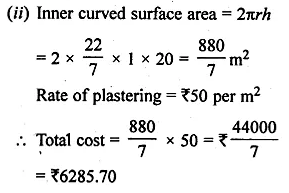Question 6.
A road roller (in the shape of a cylinder) has a diameter 0.7 m and its width is 1.2 m. Find the least number of revolutions that the roller must make in order to level a playground of size 120 m by 44 m.
Solution: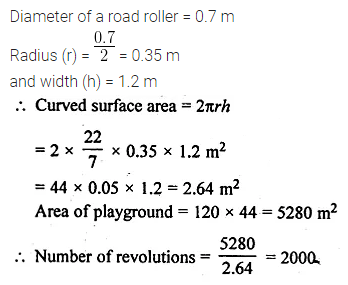Question 7.
If the volume of a cylinder of height 7 cm is 448 π cm3, find its lateral surface area and total surface area.
Solution: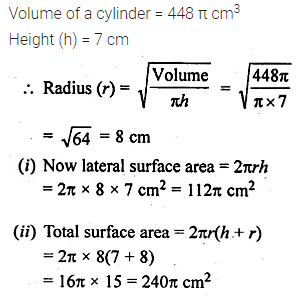Question 8.
A wooden pole is 7 m high and 20 cm in diameter. Find its weight if the wood weighs 225 kg per m3.
Solution:Question 9.
The area of the curved surface of a cylinder is 4400 cm2, and the circumference of its base is 110 cm. Find
(i) the height of the cylinder.
(ii) the volume of the cylinder.
Solution: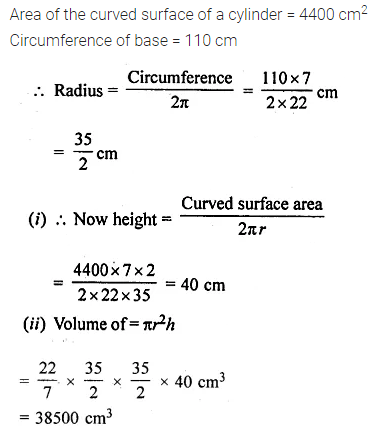Question 10.
A cylinder has a diameter of 20 cm. The area of the curved surface is 1000 cm2. Find
(i) the height of the cylinder correct to one decimal place.
(ii) the volume of the cylinder correct to one decimal place. (Take π = 3.14)
Solution: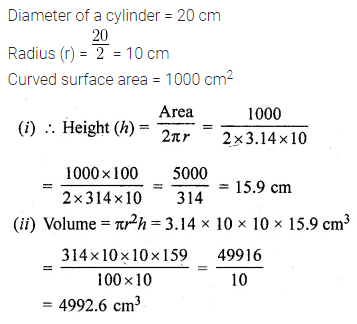Question 11.
The barrel of a fountain pen, cylindrical in shape, is 7 cm long and 5 mm in diameter. A full barrel of ink in the pen will be used up when writing 310 words on an average. How many words would use up a bottle of ink containing one-fifth of a litre?
Solution: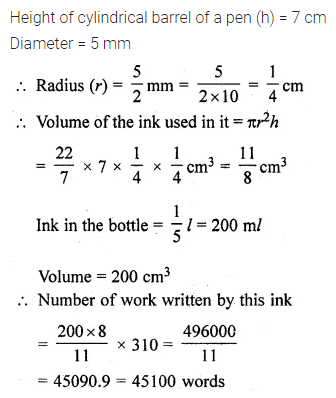Question 12.
Find the ratio between the total surface area of a cylinder to its curved surface area given that its height and radius are 7.5 cm and 3.5 cm.
Solution:Question 13.
The radius of the base of a right circular cylinder is halved and the height is doubled. What is the ratio of the volume of the new cylinder to that of the original cylinder?
Solution: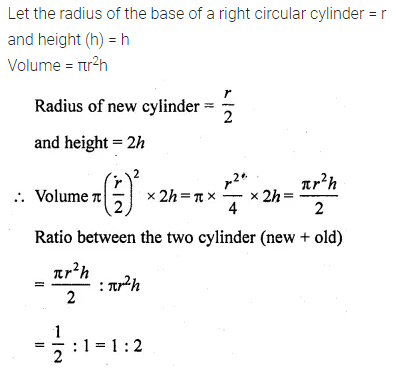Question 14.
(i) The sum of the radius and the height of a cylinder is 37 cm and the total surface area of the cylinder is 1628 cm2. Find the height and the volume of the cylinder.
(ii) The total surface area of a cylinder is 352 cm2. If its height is 10 cm, then find the diameter of the base.
Solution: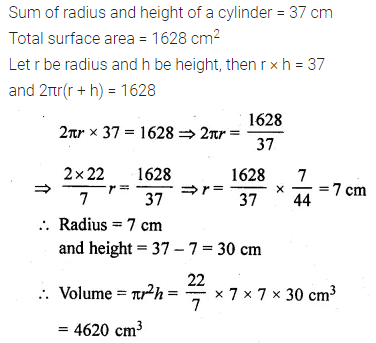Question 15.
The ratio between the curved surface and the total surface of a cylinder is 1 : 2. Find the volume of the cylinder, given that its total surface area is 616 cm2.
Solution: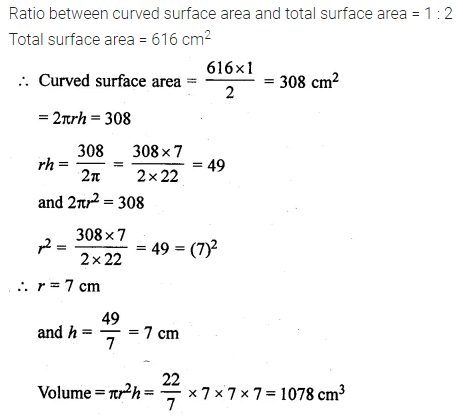Question 16.
Two cylindrical jars contain the same amount of milk. If their diameters are in the ratio 3 : 4, find the ratio of their heights.
Solution: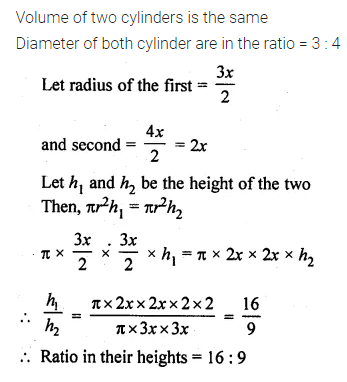Question 17.
A rectangular sheet of tin foil of size 30 cm x 18 cm can be rolled to form a cylinder in two ways along the length and along with breadth. Find the ratio of volumes of the two cylinders thus formed.
Solution:Question 18.
A cylindrical tube open at both ends is made of metal. The internal diameter of the tube is 11.2 cm and its length is 21 cm. The metal thickness is 0.4 cm. Calculate the volume of the metal.
Solution: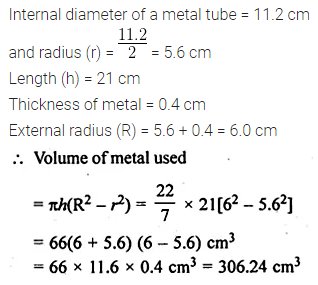Question 19.
The given figure shows a metal pipe 77 cm long. The inner diameter of a cross-section is 4 cm and the outer one is 4.4 cm. Find its
(i) inner curved surface area
(ii) outer curved surface area
(iii) total surface area.Solution: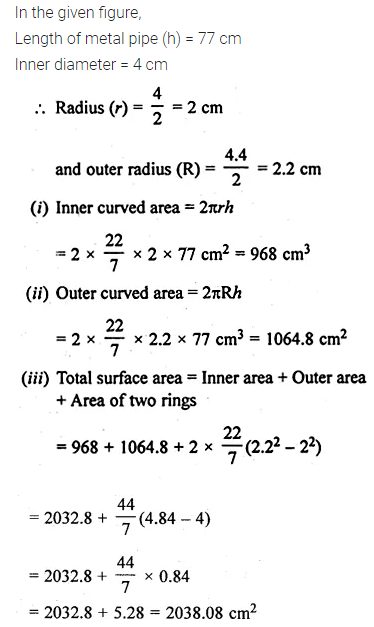Question 20.
A lead pencil consists of a cylinder of wood with a solid cylinder of graphite filled in the interior. The diameter of the pencil is 7 mm and the diameter of the graphite is 1 mm. If the length of the pencil is 14 cm, find the volume of the wood and that of the graphite.
Solution: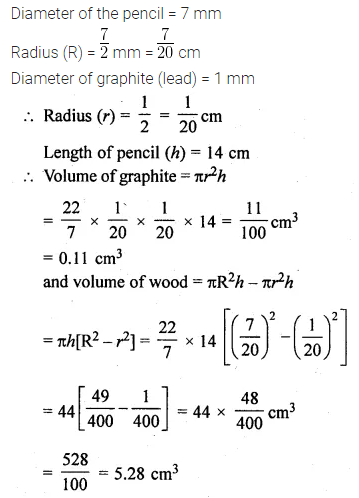Question 21.
A soft drink is available in two packs
(i) a tin can with a rectangular base of length 5 cm and width 4 cm, having a height of 15 cm and
(ii) a plastic cylinder with a circular base of diameter 7 cm and height 10 cm. Which container has greater capacity and by how much?
Solution:Question 22.
A cylindrical roller made of iron is 2 m long. Its inner diameter is 35 cm and the thickness is 7 cm all around. Find the weight of the roller in kg, if 1 cm³ of iron weighs 8 g.
Solution: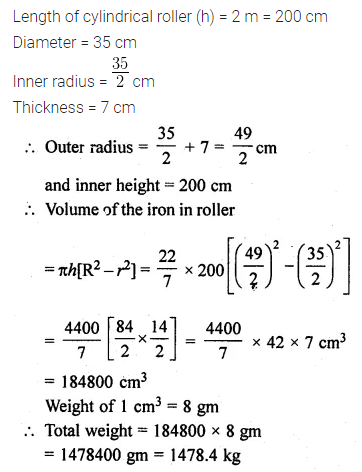ML Aggarwal Class 10 Solutions for ICSE Maths